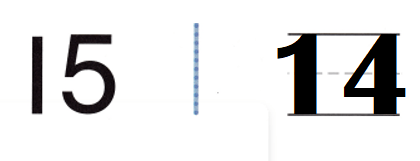# Texas Go Math Kindergarten Module 7 Assessment Answer Key

Refer to our Texas Go Math Kindergarten Answer Key Pdf to score good marks in the exams. Test yourself by practicing the problems from Texas Go Math Kindergarten Module 7 Assessment Answer Key.

## Texas Go Math Kindergarten Module 7 Assessment Answer Key

Concepts and Skills

DIRECTIONS: 1-4. Count and tell how many. Write the number. (TEKS K.2.B)

Question 1.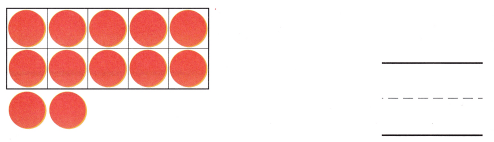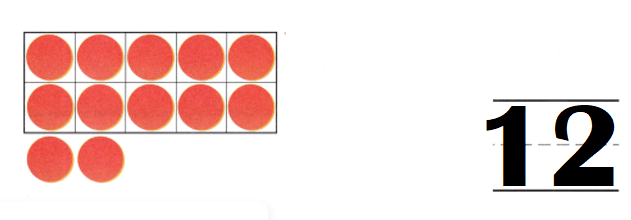Explanation:
Number of counters = 10 + 2 = 12 or Twelve.

Question 2.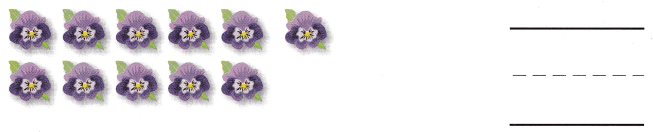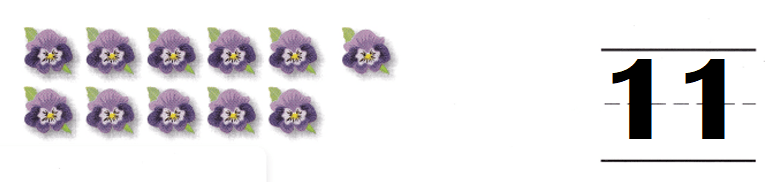Explanation:
Number of flowers = 10 + 1 = 11 or Eleven.

Question 3.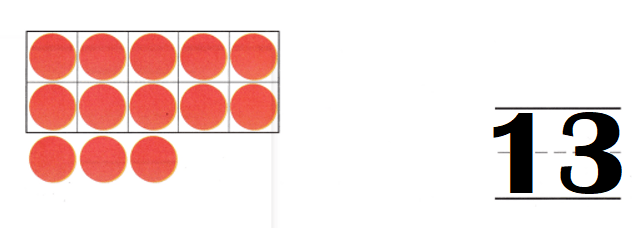Explanation:
Number of counters = 10 + 3 = 13 or Thirteen.

Question 4.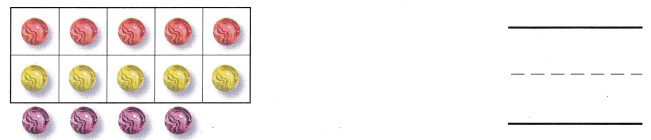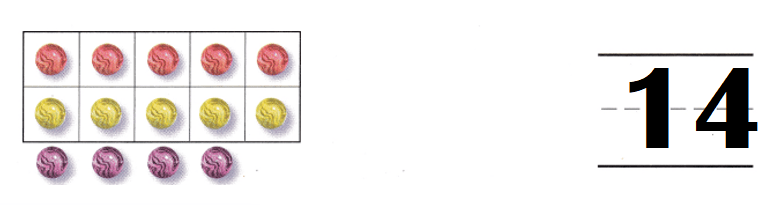Explanation:
Number of marbles = 14 or Fourteen.

DIRECTIONS: 5. Count and tell how many. Write the number. (TEKS K.2.B.) 6-7. Write the number that is one more. 8-9. Write the number that is one less. (TEKS K.2.F)

Question 5.Explanation:
Number of counters = 15 or Fifteen.

Question 6.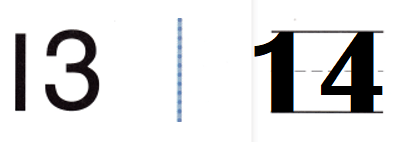Explanation:
One more than 13 = 13 + 1 = 14 or Fourteen.

Question 7.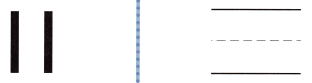Explanation:
One more than 11 = 11 + 1 = 12 or Twelve.

Question 8.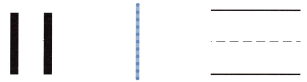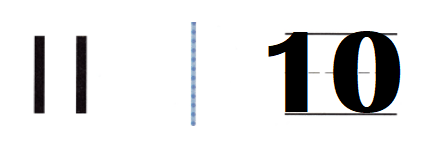Explanation:
One less than 11 = 11 – 1 = 10 or Ten.

Question 9.
Texas Test Prep